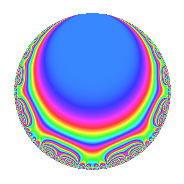# Properties

 Label 672.2.i.aLevel 672 Weight 2 Character orbit 672.i Analytic conductor 5.366 Analytic rank 0 Dimension 4 CM No Inner twists 4

# Related objects

## Newspace parameters

 Level: $$N$$ = $$672 = 2^{5} \cdot 3 \cdot 7$$ Weight: $$k$$ = $$2$$ Character orbit: $$[\chi]$$ = 672.i (of order $$2$$ and degree $$1$$)

## Newform invariants

 Self dual: No Analytic conductor: $$5.36594701583$$ Analytic rank: $$0$$ Dimension: $$4$$ Coefficient field: $$\Q(\sqrt{-2}, \sqrt{-3})$$ Coefficient ring: $$\Z[a_1, \ldots, a_{7}]$$ Coefficient ring index: $$2^{2}$$ Sato-Tate group: $\mathrm{SU}(2)[C_{2}]$

## $q$-expansion

Coefficients of the $$q$$-expansion are expressed in terms of a basis $$1,\beta_1,\beta_2,\beta_3$$ for the coefficient ring described below. We also show the integral $$q$$-expansion of the trace form.

 $$f(q)$$ $$=$$ $$q + ( -1 - \beta_{1} ) q^{3} + \beta_{1} q^{5} + ( 2 - \beta_{2} ) q^{7} + ( -1 + 2 \beta_{1} ) q^{9} +O(q^{10})$$ $$q + ( -1 - \beta_{1} ) q^{3} + \beta_{1} q^{5} + ( 2 - \beta_{2} ) q^{7} + ( -1 + 2 \beta_{1} ) q^{9} + \beta_{3} q^{11} -2 q^{13} + ( 2 - \beta_{1} ) q^{15} + 3 \beta_{3} q^{17} -4 q^{19} + ( -2 - 2 \beta_{1} + \beta_{2} - \beta_{3} ) q^{21} + \beta_{1} q^{23} + 3 q^{25} + ( 5 - \beta_{1} ) q^{27} + 2 \beta_{3} q^{29} -4 \beta_{2} q^{31} + ( -2 \beta_{2} - \beta_{3} ) q^{33} + ( 2 \beta_{1} + \beta_{3} ) q^{35} -6 \beta_{2} q^{37} + ( 2 + 2 \beta_{1} ) q^{39} -\beta_{3} q^{41} + 2 \beta_{2} q^{43} + ( -4 - \beta_{1} ) q^{45} + 2 \beta_{3} q^{47} + ( 1 - 4 \beta_{2} ) q^{49} + ( -6 \beta_{2} - 3 \beta_{3} ) q^{51} + 2 \beta_{2} q^{55} + ( 4 + 4 \beta_{1} ) q^{57} -4 \beta_{1} q^{59} + 10 q^{61} + ( -2 + 4 \beta_{1} + \beta_{2} + 2 \beta_{3} ) q^{63} -2 \beta_{1} q^{65} -2 \beta_{2} q^{67} + ( 2 - \beta_{1} ) q^{69} + \beta_{1} q^{71} + ( -3 - 3 \beta_{1} ) q^{75} + ( -3 \beta_{1} + 2 \beta_{3} ) q^{77} -8 q^{79} + ( -7 - 4 \beta_{1} ) q^{81} + 8 \beta_{1} q^{83} + 6 \beta_{2} q^{85} + ( -4 \beta_{2} - 2 \beta_{3} ) q^{87} -3 \beta_{3} q^{89} + ( -4 + 2 \beta_{2} ) q^{91} + ( 4 \beta_{2} - 4 \beta_{3} ) q^{93} -4 \beta_{1} q^{95} + 8 \beta_{2} q^{97} + ( 4 \beta_{2} - \beta_{3} ) q^{99} +O(q^{100})$$ $$\operatorname{Tr}(f)(q)$$ $$=$$ $$4q - 4q^{3} + 8q^{7} - 4q^{9} + O(q^{10})$$ $$4q - 4q^{3} + 8q^{7} - 4q^{9} - 8q^{13} + 8q^{15} - 16q^{19} - 8q^{21} + 12q^{25} + 20q^{27} + 8q^{39} - 16q^{45} + 4q^{49} + 16q^{57} + 40q^{61} - 8q^{63} + 8q^{69} - 12q^{75} - 32q^{79} - 28q^{81} - 16q^{91} + O(q^{100})$$

Basis of coefficient ring in terms of a root $$\nu$$ of $$x^{4} - 2 x^{2} + 4$$:

 $$\beta_{0}$$ $$=$$ $$1$$ $$\beta_{1}$$ $$=$$ $$\nu^{3}$$$$/2$$ $$\beta_{2}$$ $$=$$ $$\nu^{2} - 1$$ $$\beta_{3}$$ $$=$$ $$($$$$-\nu^{3} + 4 \nu$$$$)/2$$
 $$1$$ $$=$$ $$\beta_0$$ $$\nu$$ $$=$$ $$($$$$\beta_{3} + \beta_{1}$$$$)/2$$ $$\nu^{2}$$ $$=$$ $$\beta_{2} + 1$$ $$\nu^{3}$$ $$=$$ $$2 \beta_{1}$$

## Character Values

We give the values of $$\chi$$ on generators for $$\left(\mathbb{Z}/672\mathbb{Z}\right)^\times$$.

 $$n$$ $$127$$ $$421$$ $$449$$ $$577$$ $$\chi(n)$$ $$1$$ $$-1$$ $$-1$$ $$-1$$

## Embeddings

For each embedding $$\iota_m$$ of the coefficient field, the values $$\iota_m(a_n)$$ are shown below.

For more information on an embedded modular form you can click on its label.

Label $$\iota_m(\nu)$$ $$a_{2}$$ $$a_{3}$$ $$a_{4}$$ $$a_{5}$$ $$a_{6}$$ $$a_{7}$$ $$a_{8}$$ $$a_{9}$$ $$a_{10}$$
209.1
 1.22474 + 0.707107i −1.22474 + 0.707107i −1.22474 − 0.707107i 1.22474 − 0.707107i
0 −1.00000 1.41421i 0 1.41421i 0 2.00000 1.73205i 0 −1.00000 + 2.82843i 0
209.2 0 −1.00000 1.41421i 0 1.41421i 0 2.00000 + 1.73205i 0 −1.00000 + 2.82843i 0
209.3 0 −1.00000 + 1.41421i 0 1.41421i 0 2.00000 1.73205i 0 −1.00000 2.82843i 0
209.4 0 −1.00000 + 1.41421i 0 1.41421i 0 2.00000 + 1.73205i 0 −1.00000 2.82843i 0
 $$n$$: e.g. 2-40 or 990-1000 Significant digits: Format: Complex embeddings Normalized embeddings Satake parameters Satake angles

## Inner twists

Char. orbit Parity Mult. Self Twist Proved
1.a Even 1 trivial yes
3.b Odd 1 yes
56.h Odd 1 yes
168.i Even 1 yes

## Hecke kernels

This newform can be constructed as the intersection of the kernels of the following linear operators acting on $$S_{2}^{\mathrm{new}}(672, [\chi])$$:

 $$T_{5}^{2} + 2$$ $$T_{11}^{2} - 6$$ $$T_{13} + 2$$